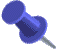## Gordon Nipp's Tables of Quaternary Quadratic FormsKeywords: tables, reduced, regular, primitive, positive definite, quaternary, quadratic forms, four-dimensional lattices, automorphism group, mass, genus, genera, Hasse symbol, Jordan splittingThese tables were computed by Gordon L. Nipp (gnipp@calstatela.edu), of the Department of Mathematics, California State University, Los Angeles, CA 90032, USA, and were published in his book: Gordon L. Nipp, "Quaternary Quadratic Forms: Computer Generated Tables", Springer-Verlag, New York, 1991; ISBN 0-387-97601-9. They are included here with his permission.

Some corrections to these tables have been found by Chul-hee Lee Minor corrections to Nipps tables of quaternary quadratic forms .
For the corrected information compiled by Chul-hee Lee see github tables.## Contents of these files## Format of these files

These are tables of reduced regular primitive positive-definite quaternary quadratic forms over the rational integers.

They were computed by Gordon L. Nipp (see above) and are included here with his permission.

The discriminant d of a quaternary quadratic form

f = f11 x1^2 + f22 x2^2 + f33 x3^2 + f44 x4^2
+ f12 x1 x2 + f13 x1 x3 + f23 x2 x3 + f14 x1 x4 + f24 x2 x4 + f34 x3 x4

where the coefficients f11, f12, ..., f44 are integers, is defined to be the determinant of the associated matrix F =

[ 2f11   f12   f13   f14 ]
[ f12   2f22   f23   f24 ]
[ f13   f23   2f33   f34 ]
[ f14   f24   f34   2f44 ]

Then d is an integer congruent to 0 or 1 mod 4.

In the discriminant tables there is one line for each form, containing 17 entries:

d   g   f11   f22   f33   f44   f12   f13   f23   f14   f24   f34   H   N   G   m1   m2

where
d = discriminant,
g = number of genus to which f belongs,
f11 ... f34 are the coefficients,
H = Hasse symbol at all primes p dividing 2d, in increasing order of p (using 10 characters),
N = level of   f = smallest N such that N F^(-1) has integer entries and even diagonal entries,
G = number of automorphisms of   f,
m1/m2 = the total mass of this genus

As a check, the sum of 1/G over all forms with the same first two entries (i.e. the same genus) should equal m1/m2

The two genera files give further information about each genus. For each discriminant d and each genus, there is a line for each prime p dividing 2d, giving the p-adic density, and a p-adic Jordan splitting for the first form listed in the genus.

See Nipp's book mentioned above for further information about these tables.